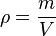# Density facts for kids

Kids Encyclopedia Facts

Density is a measurement that compares the amount of matter an object has to its volume. An object with much matter in a certain volume has high density. An object with little matter in the same amount of volume has a low density. Density is found by dividing the mass of an object by its volume.$\rho = \frac{m}{V}$

where ρ is the density, m is the mass, and V is the volume.

## Changes of density

In general, density can be changed by changing either the pressure or the temperature. Increasing the pressure always increases the density of a material. Increasing the temperature usually lowers the density, but there are exceptions. For example, the density of water increases slightly between its melting point at 0 °C and 4 °C. When water freezes, it expands by about 9% in volume, making ice that is less dense than liquid water. Water expands as it drops below 4 °C.

## Measures

Fresh water is often used as a standard of relative density. This is called specific gravity.

The most common SI units for density are g/cm3 and kg/m3. When the numerator is much larger than the denominator, that means the substance has a higher density. When the denominator is much larger than the numerator, the substance has a lower density.

## Other uses of the term

"Density" sometimes means the ratio between other properties instead of mass and volume. Then it means how much of a property can be found in a specific piece of what they are looking at. For example, population density is how many people live within the same amount of land area. The population density in the city is higher than the country side because people live closer to each other in the city. In computers, storage density is how much data can fit on a data storage device in relation to its physical size. A Blu-ray disc has a higher storage density than a DVD which has a higher storage density than a CD, even though they all have almost exactly the same volume.Density Facts for Kids. Kiddle Encyclopedia.